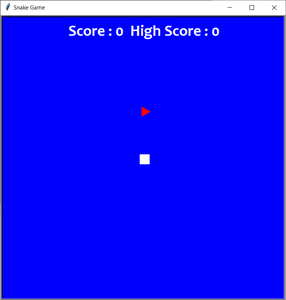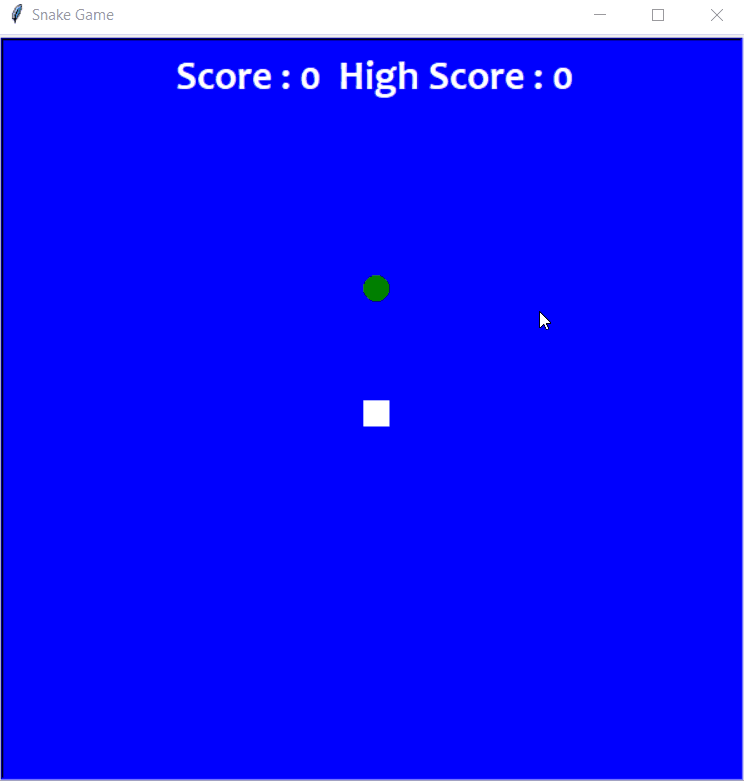GeeksforGeeks App
Open AppBrowser
Continue

# Create a Snake-Game using Turtle in Python

A snake game is an arcade maze game which has been developed by Gremlin Industries and published by Sega in October 1976. It is considered to be a skillful game and has been popularized among people for generations. The snake in the Snake game is controlled using the four direction buttons relative to the direction it is headed in. The player’s objective in the game is to achieve maximum points as possible by collecting food or fruits. The player loses once the snake hits the wall or hits itself.

For the python beginners, those who are interested in making something easier in your domain can definitely try this out and the module Turtle was made exactly for this purpose for the beginners to try out and can also submit as a part of the project. This program will be done in Python 3.

So, we will be creating a Python-based-game using the following modules:

• Turtle: It is a pre-installed python library that enables users to create shapes and pictures by providing them with a virtual canvas.
• Time: This function is used to count the number of seconds elapsed since the epoch.
• Random: This function is used to generate random numbers in Python by using random module.

### Support

The following code can be easily done using PyCharm application which is specially made for Python programs.

Also, VSCode can be used for this program. Install Python3 from extensions of VSCode. Then, save the program in the form of your_filename.py

### Below is the step-by-step Approach to create a Snake Game using Turtle module:

Step 1: We will be importing modules into the program and giving default values for the game.

## Python3

 `import` `turtle``import` `time``import` `random` `delay ``=` `0.1``score ``=` `0``high_score ``=` `0`

Step 2: Now, we will be creating the display of the game, i.e, the window screen for the game where we will create the head of the snake and food for the snake in the game and displaying the scores at the header of the game.

## Python3

 `# Creating a window screen``wn ``=` `turtle.Screen()``wn.title(``"Snake Game"``)``wn.bgcolor(``"blue"``)` `# the width and height can be put as user's choice``wn.setup(width``=``600``, height``=``600``)``wn.tracer(``0``)`  `# head of the snake``head ``=` `turtle.Turtle()``head.shape(``"square"``)``head.color(``"white"``)``head.penup()``head.goto(``0``, ``0``)``head.direction ``=` `"Stop"`  `# food in the game``food ``=` `turtle.Turtle()``colors ``=` `random.choice([``'red'``, ``'green'``, ``'black'``])``shapes ``=` `random.choice([``'square'``, ``'triangle'``, ``'circle'``])``food.speed(``0``)``food.shape(shapes)``food.color(colors)``food.penup()``food.goto(``0``, ``100``)`  `pen ``=` `turtle.Turtle()``pen.speed(``0``)``pen.shape(``"square"``)``pen.color(``"white"``)``pen.penup()``pen.hideturtle()``pen.goto(``0``, ``250``)``pen.write(``"Score : 0  High Score : 0"``, align``=``"center"``,``          ``font``=``(``"candara"``, ``24``, ``"bold"``))`

Output:Step 3: Now, we will be validating the key for the snake’s movements. By clicking the keywords normally used for gaming ‘w’, ‘a’, ‘s’ and ‘d’, we can operate the snake’s movements around the screen.

## Python3

 `# assigning key directions``def` `group():``    ``if` `head.direction !``=` `"down"``:``        ``head.direction ``=` `"up"`  `def` `godown():``    ``if` `head.direction !``=` `"up"``:``        ``head.direction ``=` `"down"`  `def` `goleft():``    ``if` `head.direction !``=` `"right"``:``        ``head.direction ``=` `"left"`  `def` `goright():``    ``if` `head.direction !``=` `"left"``:``        ``head.direction ``=` `"right"`  `def` `move():``    ``if` `head.direction ``=``=` `"up"``:``        ``y ``=` `head.ycor()``        ``head.sety(y``+``20``)``    ``if` `head.direction ``=``=` `"down"``:``        ``y ``=` `head.ycor()``        ``head.sety(y``-``20``)``    ``if` `head.direction ``=``=` `"left"``:``        ``x ``=` `head.xcor()``        ``head.setx(x``-``20``)``    ``if` `head.direction ``=``=` `"right"``:``        ``x ``=` `head.xcor()``        ``head.setx(x``+``20``)`  `wn.listen()``wn.onkeypress(group, ``"w"``)``wn.onkeypress(godown, ``"s"``)``wn.onkeypress(goleft, ``"a"``)``wn.onkeypress(goright, ``"d"``)`

Step 4: Now, lastly, we will create the gameplay where the following will be happening:

• The snake will grow its body when the snake eats the fruits.
• Giving color to the snake’s tail.
• After the fruit is eaten, the score will be counted.
• Checking for the snake’s head collisions with the body or the wall of the window screen.
• Restarting the game automatically from the start after the collision.
• The new shape and color of the fruit will be introduced every time the window is restarted.
• The score will be returned to zero and a high score will be retained until the window is not closed.

## Python3

 `segments ``=` `[]` `# Main Gameplay``while` `True``:``    ``wn.update()``    ``if` `head.xcor() > ``290` `or` `head.xcor() < ``-``290` `or` `head.ycor() > ``290` `or` `head.ycor() < ``-``290``:``        ``time.sleep(``1``)``        ``head.goto(``0``, ``0``)``        ``head.direction ``=` `"Stop"``        ``colors ``=` `random.choice([``'red'``, ``'blue'``, ``'green'``])``        ``shapes ``=` `random.choice([``'square'``, ``'circle'``])``        ``for` `segment ``in` `segments:``            ``segment.goto(``1000``, ``1000``)``        ``segments.clear()``        ``score ``=` `0``        ``delay ``=` `0.1``        ``pen.clear()``        ``pen.write(``"Score : {} High Score : {} "``.``format``(``            ``score, high_score), align``=``"center"``, font``=``(``"candara"``, ``24``, ``"bold"``))``    ``if` `head.distance(food) < ``20``:``        ``x ``=` `random.randint(``-``270``, ``270``)``        ``y ``=` `random.randint(``-``270``, ``270``)``        ``food.goto(x, y)` `        ``# Adding segment``        ``new_segment ``=` `turtle.Turtle()``        ``new_segment.speed(``0``)``        ``new_segment.shape(``"square"``)``        ``new_segment.color(``"orange"``)  ``# tail colour``        ``new_segment.penup()``        ``segments.append(new_segment)``        ``delay ``-``=` `0.001``        ``score ``+``=` `10``        ``if` `score > high_score:``            ``high_score ``=` `score``        ``pen.clear()``        ``pen.write(``"Score : {} High Score : {} "``.``format``(``            ``score, high_score), align``=``"center"``, font``=``(``"candara"``, ``24``, ``"bold"``))``    ``# Checking for head collisions with body segments``    ``for` `index ``in` `range``(``len``(segments)``-``1``, ``0``, ``-``1``):``        ``x ``=` `segments[index``-``1``].xcor()``        ``y ``=` `segments[index``-``1``].ycor()``        ``segments[index].goto(x, y)``    ``if` `len``(segments) > ``0``:``        ``x ``=` `head.xcor()``        ``y ``=` `head.ycor()``        ``segments[``0``].goto(x, y)``    ``move()``    ``for` `segment ``in` `segments:``        ``if` `segment.distance(head) < ``20``:``            ``time.sleep(``1``)``            ``head.goto(``0``, ``0``)``            ``head.direction ``=` `"stop"``            ``colors ``=` `random.choice([``'red'``, ``'blue'``, ``'green'``])``            ``shapes ``=` `random.choice([``'square'``, ``'circle'``])``            ``for` `segment ``in` `segments:``                ``segment.goto(``1000``, ``1000``)``            ``segments.clear()` `            ``score ``=` `0``            ``delay ``=` `0.1``            ``pen.clear()``            ``pen.write(``"Score : {} High Score : {} "``.``format``(``                ``score, high_score), align``=``"center"``, font``=``(``"candara"``, ``24``, ``"bold"``))``    ``time.sleep(delay)` `wn.mainloop()`

Below the complete program based on the above approach:

## Python3

 `# import required modules``import` `turtle``import` `time``import` `random` `delay ``=` `0.1``score ``=` `0``high_score ``=` `0`  `# Creating a window screen``wn ``=` `turtle.Screen()``wn.title(``"Snake Game"``)``wn.bgcolor(``"blue"``)``# the width and height can be put as user's choice``wn.setup(width``=``600``, height``=``600``)``wn.tracer(``0``)` `# head of the snake``head ``=` `turtle.Turtle()``head.shape(``"square"``)``head.color(``"white"``)``head.penup()``head.goto(``0``, ``0``)``head.direction ``=` `"Stop"` `# food in the game``food ``=` `turtle.Turtle()``colors ``=` `random.choice([``'red'``, ``'green'``, ``'black'``])``shapes ``=` `random.choice([``'square'``, ``'triangle'``, ``'circle'``])``food.speed(``0``)``food.shape(shapes)``food.color(colors)``food.penup()``food.goto(``0``, ``100``)` `pen ``=` `turtle.Turtle()``pen.speed(``0``)``pen.shape(``"square"``)``pen.color(``"white"``)``pen.penup()``pen.hideturtle()``pen.goto(``0``, ``250``)``pen.write(``"Score : 0  High Score : 0"``, align``=``"center"``,``          ``font``=``(``"candara"``, ``24``, ``"bold"``))`  `# assigning key directions``def` `group():``    ``if` `head.direction !``=` `"down"``:``        ``head.direction ``=` `"up"`  `def` `godown():``    ``if` `head.direction !``=` `"up"``:``        ``head.direction ``=` `"down"`  `def` `goleft():``    ``if` `head.direction !``=` `"right"``:``        ``head.direction ``=` `"left"`  `def` `goright():``    ``if` `head.direction !``=` `"left"``:``        ``head.direction ``=` `"right"`  `def` `move():``    ``if` `head.direction ``=``=` `"up"``:``        ``y ``=` `head.ycor()``        ``head.sety(y``+``20``)``    ``if` `head.direction ``=``=` `"down"``:``        ``y ``=` `head.ycor()``        ``head.sety(y``-``20``)``    ``if` `head.direction ``=``=` `"left"``:``        ``x ``=` `head.xcor()``        ``head.setx(x``-``20``)``    ``if` `head.direction ``=``=` `"right"``:``        ``x ``=` `head.xcor()``        ``head.setx(x``+``20``)`  `wn.listen()``wn.onkeypress(group, ``"w"``)``wn.onkeypress(godown, ``"s"``)``wn.onkeypress(goleft, ``"a"``)``wn.onkeypress(goright, ``"d"``)` `segments ``=` `[]`  `# Main Gameplay``while` `True``:``    ``wn.update()``    ``if` `head.xcor() > ``290` `or` `head.xcor() < ``-``290` `or` `head.ycor() > ``290` `or` `head.ycor() < ``-``290``:``        ``time.sleep(``1``)``        ``head.goto(``0``, ``0``)``        ``head.direction ``=` `"Stop"``        ``colors ``=` `random.choice([``'red'``, ``'blue'``, ``'green'``])``        ``shapes ``=` `random.choice([``'square'``, ``'circle'``])``        ``for` `segment ``in` `segments:``            ``segment.goto(``1000``, ``1000``)``        ``segments.clear()``        ``score ``=` `0``        ``delay ``=` `0.1``        ``pen.clear()``        ``pen.write(``"Score : {} High Score : {} "``.``format``(``            ``score, high_score), align``=``"center"``, font``=``(``"candara"``, ``24``, ``"bold"``))``    ``if` `head.distance(food) < ``20``:``        ``x ``=` `random.randint(``-``270``, ``270``)``        ``y ``=` `random.randint(``-``270``, ``270``)``        ``food.goto(x, y)` `        ``# Adding segment``        ``new_segment ``=` `turtle.Turtle()``        ``new_segment.speed(``0``)``        ``new_segment.shape(``"square"``)``        ``new_segment.color(``"orange"``)  ``# tail colour``        ``new_segment.penup()``        ``segments.append(new_segment)``        ``delay ``-``=` `0.001``        ``score ``+``=` `10``        ``if` `score > high_score:``            ``high_score ``=` `score``        ``pen.clear()``        ``pen.write(``"Score : {} High Score : {} "``.``format``(``            ``score, high_score), align``=``"center"``, font``=``(``"candara"``, ``24``, ``"bold"``))``    ``# Checking for head collisions with body segments``    ``for` `index ``in` `range``(``len``(segments)``-``1``, ``0``, ``-``1``):``        ``x ``=` `segments[index``-``1``].xcor()``        ``y ``=` `segments[index``-``1``].ycor()``        ``segments[index].goto(x, y)``    ``if` `len``(segments) > ``0``:``        ``x ``=` `head.xcor()``        ``y ``=` `head.ycor()``        ``segments[``0``].goto(x, y)``    ``move()``    ``for` `segment ``in` `segments:``        ``if` `segment.distance(head) < ``20``:``            ``time.sleep(``1``)``            ``head.goto(``0``, ``0``)``            ``head.direction ``=` `"stop"``            ``colors ``=` `random.choice([``'red'``, ``'blue'``, ``'green'``])``            ``shapes ``=` `random.choice([``'square'``, ``'circle'``])``            ``for` `segment ``in` `segments:``                ``segment.goto(``1000``, ``1000``)``            ``segments.clear()` `            ``score ``=` `0``            ``delay ``=` `0.1``            ``pen.clear()``            ``pen.write(``"Score : {} High Score : {} "``.``format``(``                ``score, high_score), align``=``"center"``, font``=``(``"candara"``, ``24``, ``"bold"``))``    ``time.sleep(delay)` `wn.mainloop()`

Output:Demonstrating the final game displaying everything written in code

Code Explanation:

1. The code starts by creating a window screen.
2. The title of the window is “Snake Game”.
3. The background color of the window is blue.
4. Next, the code creates two turtle objects: head and food.
5. Head is used to control the snake, while food will be used as the game’s object.
6. The code first sets up head so that it looks like a square with white color and starts at (0, 0).
7. Next, it sets up food so that it looks like a square with blue color and places it at (10, 10).
8. Finally, head moves towards food using direction=”Stop”.
9. The code creates a window screen with the title “Snake Game” and sets the background color to blue.
10. Next, a square head object is created and set to have the color white.
11. The pen up() method is called on the head object, which causes it to rise up from the bottom of the screen.
12. Finally, the goTo() method is used to move the head object towards (0, 0) in the x-direction.
13. Next, two turtle objects are created – one for food and one for head.
14. The food object has a shape of “square” and will be placed at (0, 0) on the screen.
15. The head object has no shape specified and will be placed at (0, 0) in the y-direction
16. The code starts by creating a few variables to store information about the game.
17. The first is food, which stores information about the shapes and colors of the food that will be displayed on the screen.
18. Next, the code creates a function called speed() that will control how quickly the food moves across the screen.
19. Finally, the shape() and color() functions are used to create different types of food (square, triangle, and circle) with corresponding colors and speeds.
20. The next section of code sets up an event handler for when the user clicks on one of the buttons in the toolbar.
21. This handler calls a function called goto() that takes two arguments: position (in pixels) and direction (which can be “up”, “down”, “left”, or “right”).
22. goTo() then uses these values to move the pen object to a specific location onscreen and set its properties accordingly.
23. The last section of code displays some text onscreen and starts timing it using time().
24. Then it prints out a score value for each round played as well as high scores for both players at once.
25. The code will create a turtle that will move at 0 speed and have a square shape.
26. The turtle will be white in color and will start at the top left corner of the screen.
27. Once the code has executed, it will output “Score : 0 High Score : 0” in center alignment.
28. The code starts by creating three variables: head, wn, and godown.
29. The head variable stores information about the player’s current position on the screen (xcor and ycor), while wn is a listening object that will be used to track keyboard input.
30. Godown stores information about where the player should go next (if they are moving left or right), and goleft and goright store key directions for moving up, down, or left/right respectively.
31. The code then starts to loop through each of these variables in turn.
32. If any of them change (head.direction, wn.listen(), godown.direction), the code updates itself accordingly and sets various properties on head such as its xcor and ycor coordinates, its heading direction (up, down, left/right), as well as colors and shapes for each segment in the game world according to what was just inputted by the user via keyboard presses.
33. Finally, there is a delay timer set to 0.1 seconds which allows time for one frame of animation before starting over again with another round of gameplay; this is important because it ensures that everything looks smooth even when there are lots of objects moving around on-screen at once!
34. The code assigns key directions to the turtle, listens for user input, updates the screen and calculates the player’s score.
35. It also creates a new square segment if needed and moves it according to the key presses.
36. If the player is moving their turtle up or down, then head.direction will be set to “up” or “down”, respectively.
37. If they are moving left or right, head.direction will be set to “left” or “right”.
38. The code also checks whether the player’s current position falls within a food zone and if not, then the appropriate x and y coordinates are randomly generated and stored in food and moved accordingly by calling Turtle().goto() with those values.

My Personal Notes arrow_drop_up